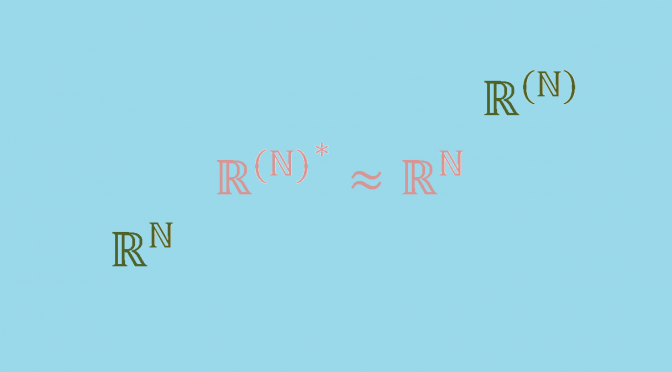# A vector space not isomorphic to its double dual

In this page $$\mathbb{F}$$ refers to a field. Given any vector space $$V$$ over $$\mathbb{F}$$, the dual space $$V^*$$ is defined as the set of all linear functionals $$f: V \mapsto \mathbb{F}$$. The dual space $$V^*$$ itself becomes a vector space over $$\mathbb{F}$$ when equipped with the following addition and scalar multiplication:
$\left\{ \begin{array}{lll}(\varphi + \psi)(x) & = & \varphi(x) + \psi(x) \\ (a \varphi)(x) & = & a (\varphi(x)) \end{array} \right.$ for all $$\phi, \psi \in V^*$$, $$x \in V$$, and $$a \in \mathbb{F}$$.
There is a natural homomorphism $$\Phi$$ from $$V$$ into the double dual $$V^{**}$$, defined by $$(\Phi(v))(\phi) = \phi(v)$$ for all $$v \in V$$, $$\phi \in V^*$$. This map $$\Phi$$ is always injective.

## Finite-dimensional case

Given a basis $$\{\textbf{e}_1, \dots, \textbf{e}_n\}$$ in $$V$$, it is possible to construct a specific basis in $$V^*$$, called the dual basis. This dual basis is a set $$\{\textbf{e}^*_1,\dots,\textbf{e}^*_n\}$$ of linear functionals on $$V$$, defined by the relations:
$\mathbf{e}^*_i(\mathbf{e}_j) = \delta^{i}_{j} \mbox{ for } 1 \leq i \leq n, 1 \leq j \leq n$ where $$\delta^{i}_{j}$$ is the Kronecker delta symbol.
Therefore, if $$V$$ is finite-dimensional, $$\dim V^{**} = \dim V^* = \dim V$$ and $$\Phi$$ is an isomorphism.

## A counter-example in the infinite-dimensional case

Let $$E=\mathbb{F}^{(\mathbb{N})}$$ denote the vector space of sequences of elements of $$\mathbb{F}$$ that are eventually $$0$$ and $$(e_i)_{n \in \mathbb{N}}$$ its canonical basis. Also let $$V=\mathbb{F}^{\mathbb{N}}$$ denote the vector space of sequences of elements of $$\mathbb{F}$$. For $$v=(v_n) \in V$$, let define $$\varphi_v \in E^*$$ by:
$\varphi_v(a)= \sum_{n=0}^\infty a_n v_n$ for $$a=(a_n) \in E$$ (the sum exists as $$a=(a_n)$$ is eventually $$0$$).
The map:
$\Theta : \left| \begin{array}{ll} V & \longrightarrow E^*\\ v & \longmapsto \varphi_v \end{array} \right.$ is an injective linear map. On the other hand, let’s take $$\varphi \in E^*$$ and note $$u_n=\varphi(e_n)$$ for $$n \in \mathbb{N}$$. We have $$\varphi = \varphi_u$$ with $$u=(u_n)$$. Which proves that $$\Theta$$ is an isomorphism.
Assuming axiom of choice, and taking the field of real numbers as $$\mathbb{F}$$, $$V$$ has a basis and has uncountable dimension, whereas $$E$$ has countable dimension.
So $$\dim E < \dim E^* = \dim V \leq \dim E^{**}$$ and $$E$$ cannot be isomorphic to its double-dual. In fact, assuming axiom of choice an infinite-dimension vector space is never isomorph to its double dual.